×#### Thank you for registering.

One of our academic counsellors will contact you within 1 working day.

Click to Chat

1800-1023-196

+91-120-4616500

CART 0

• 0

MY CART (5)

Use Coupon: CART20 and get 20% off on all online Study Material

ITEM
DETAILS
MRP
DISCOUNT
FINAL PRICE
Total Price: Rs.

There are no items in this cart.
Continue Shopping• Complete JEE Main/Advanced Course and Test Series
• OFFERED PRICE: Rs. 15,900
• View Details

```Chapter 8: Quadratic Equations Exercise – 8.5

Question: 1

Find the discriminant of the following quadratic equations:

2x2 - 5x + 3 = 0

Solution:

2x2 - 5x + 3 = 0

The given equation is in the form of ax2 + bx + c = 0

Here, a = 2, b = -5 and c = 3

The discriminant, D = b2 - 4ac

D = (-5)2 – 4 x 2 x 3

D = 25 – 24 = 1

Therefore, the discriminant of the following quadratic equation is 1.

Question: 2

Find the discriminant of the quadratic equations.

x2 + 2x + 4 = 0

Solution:

x2 + 2x + 4 = 0

The given equation is in the form of ax2 + bx + c = 0

Here, a = 1, b = 2 and c = 4

The discriminant is:-

D = (2)2 - 4 x 1 x 4

D = 4 – 16 = - 12

The discriminant of the following quadratic equation is = - 12.

Question: 3

Find the discriminant of the quadratic equations.

(x -1) (2x -1) = 0

Solution:

(x -1) (2x -1) = 0

The provided equation is (x -1) (2x -1) = 0

By solving it, we get 2x2 - 3x + 1 = 0

Now this equation is in the form of ax2 + bx + c = 0

Here, a = 2, b = -3, c = 1

The discriminant is:-

D = (-3)2 - 4 x 2 x 1

D = 9 - 8 = 1

The discriminant of the following quadratic equation is = 1.

Question: 4

Find the discriminant of the quadratic equations.

x2 - 2x + k = 0

Solution:

x2 - 2x + k = 0

The given equation is in the form of ax2 + bx + c = 0

Here, a = 1, b = -2, and c = k

D = b2 - 4ac

D = (-2)2 - 4(1)(k)

= 4 - 4k

Therefore, the discriminant, D of the equation is (4 - 4k)

Question: 5

Find the discriminant of the quadratic equations.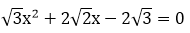Solution:The given equation is in the form of ax2 + bx + c = 0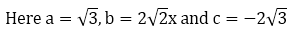The discriminant is, D = b2 - 4ac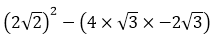D = 8 + 24 = 32

The discriminant, D of the following equation is 32.

Question: 6

Find the discriminant of the quadratic equations.

x2 - x + 1 = 0

Solution:

x2 - x + 1 = 0

The given equation is in the form of ax2 + bx + c = 0

Here, a = 1, b = -1 and c = 1

The discriminant is D = b2 - 4ac

(-1)2 - 4 × 1 × 1

1 - 4 = - 3

Therefore, the discriminant D of the following equation is -3.

Question: 7

Find the discriminant of the quadratic equations.

x2 + x + 2 = 0

Solution:

x2 + x + 2 = 0

The given equation can be written in the form of, ax2 + bx + c = 0

The discriminant is given by the following equation, D = b2 - 4ac

Here, a = 1, b = 1 and c = 2.

Therefore, the discriminant is given as,

D = (1)2 - 4(1)(2)

= 1 - 8

= - 7

For a quadratic equation to have real roots, D ≥ 0.

Here we find that the equation does not satisfy this condition, hence it does not have real roots.

Question: 8

Find the discriminant of the quadratic equations.

16x2 = 24x + 1

Solution:

16x2 - 24x - 1 = 0

The given equation can be written in the form of, ax2 + bx + c = 0

The discriminant is given by the following equation, D = b2 - 4ac

Here, a = 16, b = -24 and c = - 1

Therefore, the discriminant is given as,

D = (-24)2 - 4(16)(-1)

= 576 + 64

= 640

For a quadratic equation to have real roots, D ≥ 0.

Here it can be seen that the equation satisfies this condition, hence it has real roots.

The roots of an equation can be found out by using,Therefore, the roots of the following equation are as follows,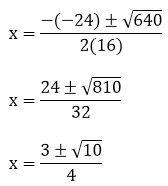The values of x for both the cases will be: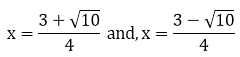Question: 9

Find the discriminant of the quadratic equations.

x2 + x + 2 = 0

Solution:

x2 + x + 2 = 0

Here, a = 1, b = 1 and c = 2.

Therefore, the discriminant is given as,

D = (1)2 - 4(1)(2)

= 1 - 8

= - 7

For a quadratic equation to have real roots, D ≥ 0.

Here we find that the equation does not satisfy this condition, hence it does not have real roots.

Question: 10

Find the discriminant of the quadratic equations.Solution:The given equation can be written in the form of, ax2 + bx + c = 0

The discriminant is given by the following equation, D = b2 - 4ac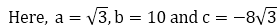Therefore, the discriminant is given as,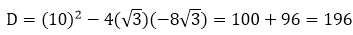For a quadratic equation to have real roots, D ≥ 0

Here it can be seen that the equation satisfies this condition, hence it has real roots.

The roots of an equation can be found out by using,Therefore, the roots of the equation are given as follows,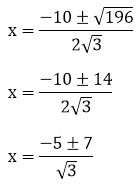The values of x for both the cases will be:Question: 11

Find the discriminant of the quadratic equations.

3x2 - 2x + 2 = 0

Solution:

3x2 - 2x + 2 = 0

The given equation can be written in the form of, ax2 + bx + c = 0

The discriminant is given by the following equation, D = b2 - 4ac

Here, a = 3, b = -2 and c = 2.

Therefore, the discriminant is given as,

D = (-2)2 - 4(3)(2)

= 4 - 24 = – 20

For a quadratic equation to have real roots, D ≥ 0

Here it can be seen that the equation does not satisfy this condition, hence it has no real roots.

Question: 12

Find the discriminant of the quadratic equations.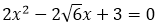Solution:The given equation can be written in the form of, ax2 + bx + c = 0

The discriminant is given by the following equation, D = b2 - 4acTherefore, the discriminant is given as,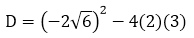= 24 - 24 = 0

For a quadratic equation to have real roots, D ≥ 0

Here it can be seen that the equation satisfies this condition, hence it has real roots.

The roots of an equation can be found out by using,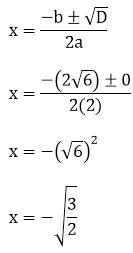Question: 13

Find the discriminant of the quadratic equations.

3a2x2 + 8abx + 4b2 = 0

Solution:

3a2x2 + 8abx + 4b2 = 0

The given equation can be written in the form of, ax2 +  bx + c = 0

The discriminant is given by the following equation, D = b2 - 4ac

Here, a = 3a2, b = 8ab and c = 4b2

Therefore, the discriminant is given as,

D = (8ab)2 - 4(3a2)(4b2)

= 64a2b2 - 48a2b2 = 16a2b2

For a quadratic equation to have real roots, D ≥ 0

Here it can be seen that the equation satisfies this condition, hence it has real roots.

The roots of an equation can be found out by using,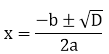Therefore, the roots of the equation are given as follows,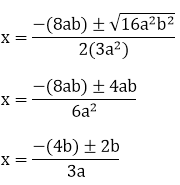The values of x for both the cases will be:Question: 14

Find the discriminant of the quadratic equations.Solution:The given equation can be written in the form of, ax2 + bx + c = 0

The discriminant is given by the following equation, D = b2 - 4ac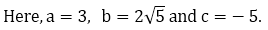Therefore, the discriminant is given as,= 20 + 60

= 80

For a quadratic equation to have real roots, D ≥ 0

Here it can be seen that the equation satisfies this condition, hence it has real roots.

The roots of an equation can be found out by using,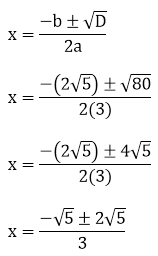The values of x for both the cases will be: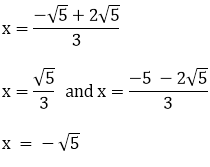Question: 15

Find the discriminant of the quadratic equations.

x2 - 2x + 1 = 0

Solution:

x2 - 2x + 1 = 0

The given equation can be written in the form of, ax2 + bx + c = 0

The discriminant is given by the following equation, D = b2 - 4ac

Here, a =1, b = - 2 and c = 1

Therefore, the discriminant is given as,

D = (-2)2 - 4(1)(1)

= 4 - 4

= 0

For a quadratic equation to have real roots, D ≥ 0

Here it can be seen that the equation satisfies this condition, hence it has real roots.

The roots of an equation can be found out by using,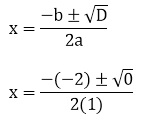x = 2/2

x = 1

Therefore, the equation real roots and its value is 1

Question: 16

Find the discriminant of the quadratic equations.Solution:The given equation can be written in the form of, ax2 + bx + c = 0

The discriminant is given by the following equation, D = b2 - 4ac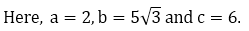Therefore, the discriminant is given as,= 75 - 48

= 27

For a quadratic equation to have real roots, D ≥ 0

Here it can be seen that the equation satisfies this condition, hence it has real roots.

The roots of an equation can be found out by using,\Therefore, the roots of the equation are given as follows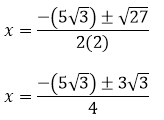The values of x for both the cases will be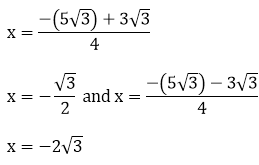Question: 17

Find the discriminant of the quadratic equations.Solution:The given equation can be written in the form of, ax2 + bx + c = 0

The discriminant is given by the following equation, D = b2 - 4ac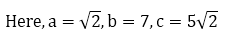Therefore, the discriminant is given as,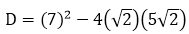D = 49 - 40

D = 9

For a quadratic equation to have real roots, D ≥ 0

Here it can be seen that the equation satisfies this condition, hence it has real roots.

The roots of an equation can be found out by using,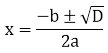Therefore, the roots of the equation are given as follows,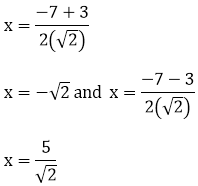Question: 18

Find the discriminant of the quadratic equations.Solution:The given equation can be written in the form of, ax2 + bx + c = 0

The discriminant is given by the following equation, D = b2 - 4ac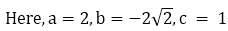Therefore, the discriminant is given as,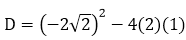= 8 - 8

= 0

For a quadratic equation to have real roots, D ≥ 0

Here it can be seen that the equation satisfies this condition, hence it has real roots.

The roots of an equation can be found out by using,Therefore, the roots of the equation are given as follows,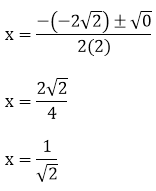Question: 19

Find the discriminant of the quadratic equations.

3x2 - 5x + 2 = 0

Solution:

3x2 - 5x + 2 = 0

The given equation can be written in the form of, ax2 + bx + c = 0

The discriminant is given by the following equation, D = b2 - 4ac

Here, a = 3, b = -5 and c = 2.

Therefore, the discriminant is given as,

D = (-5)2 - 4(3)(2)

= 25 - 24

= 1

For a quadratic equation to have real roots, D ≥ 0

Here it can be seen that the equation satisfies this condition, hence it has real roots.

The roots of an equation can be found out by using,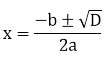Therefore, the roots of the equation are given as follows,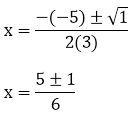The values of x for both the cases will be: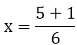x = 1

And,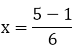x = 2/3

Question: 20

Solve for x:Solution:The above equation can be solved as follows: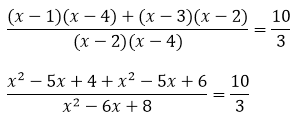6x2 - 30x + 30 = 10x2 - 60x +80

4x2 - 30x + 50 = 0

2x2 - 15x + 25 = 0

The above equation is in the form of ax2 + bx + c = 0

The discriminant is given by the equation, D = b2 - 4ac

Here, a = 2, b = -15, c = 25

D = (-15)2 - 4(2)(25)

= 225 - 200

= 25

The roots of an equation can be found out by using,Therefore, the roots of the equation are given as follows,The values of x for both the cases will be:x = 5

Also,x = 5/2

Question: 21

Solve for x:Solution:The above equation can be solved as follows: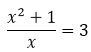x2 + 1 = 3x

x2 - 3x + 1 = 0

The above equation is in the form of ax2 + bx + c = 0

The discriminant is given by the equation, D = b2 - 4ac

Here, a = 1, b = - 3, c = 1

D = (-3)2 - 4(1)(1)

D = 9 - 4

D = 5

The roots of an equation can be found out by using,The values of x for both the cases will be:Question: 22

Solve for x: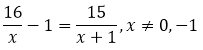Solution:The above equation can be solved as follows: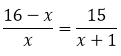(16 - x)(x + 1) = 15x

16x + 16 - x2 - x = 15x

15x + 16 - x2 - 15x = 0

16 - x2 = 0

X2 - 16 = 0

The above equation is in the form of ax2 + bx + c = 0

The discriminant is given by the equation, D = b2 - 4ac

Here, a = 1, b = 0, c = -16

D = (0)2 - 4(1)(-16)

D = 64

The roots of an equation can be found out by using,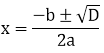Therefore, the roots of the equation are given as follows,```### Course Features

• 728 Video Lectures
• Revision Notes
• Previous Year Papers
• Mind Map
• Study Planner
• NCERT Solutions
• Discussion Forum
• Test paper with Video Solution